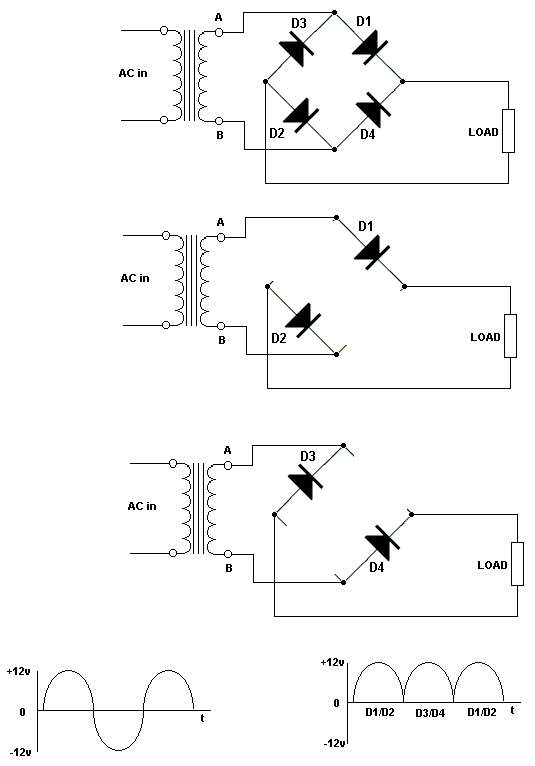Electronic Circuits and Tutorials
Tutorials
Dictionaries
Projects
Discover
Home > Electronics Tutorial > The Diode Tutorial > Full Wave Rectifier Tutorial
The Diode - Electronic Tutorials

# Full Wave Rectifier TutorialThe waveform shows the input voltage (50 Hz AC in the UK). The voltages at points A and B on the transformer are changing in opposite directions. When A is increasing in a positive direction, B is increasing negatively. It is like the opposite ends of a see-saw.

During the first half cycle, A is positive and B is negative. D1 has positive on its anode, D2 has negative on its cathode. Both are forward biased. Current flows around the circuit formed by these diodes, the load and the transformer winding, as shown in the second diagram. The current flowing up through the load produces a pulse of voltage across the load as shown in the right hand waveform.

During the next half cycle, A is negative and B is positive. D4 has positive on its anode, D3 has negative on its cathode. Both are forward biased. Current flows around the circuit as shown in the bottom diagram, again flowing in the same direction through the load and producing another pulse of voltage.

Since the full cycle is used this circuit is called a FULL-WAVE rectifier.

Since there are two pulses for each cycle of input, there are 100 pulses per second out (in the UK).

The pulsating DC can be smoothed with a reservoir capacitor, as in the half-wave rectifier circuit.
Since the pulse frequency is higher than that of the half-wave rectifier, it is easier to smooth.